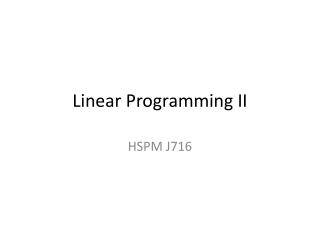Download PresentationLinear Programming II# Linear Programming II - PowerPoint PPT Presentation

Download Presentation##### Linear Programming II

Download Policy: Content on the Website is provided to you AS IS for your information and personal use and may not be sold / licensed / shared on other websites without getting consent from its author. While downloading, if for some reason you are not able to download a presentation, the publisher may have deleted the file from their server.

- - - - - - - - - - - - - - - - - - - - - - - - - - - E N D - - - - - - - - - - - - - - - - - - - - - - - - - - -
##### Presentation Transcript

1. Linear Programming II HSPM J716

2. Crawling along the simplex • The simplex method moves from one corner to the next until the amount to be maximized stops rising.

3. Learning Objectives • Learning Objective 1: Recognize problems that linear programming can handle: • Linear programming lets you optimize an objective function subject to some constraints. The objective function and constraints are all linear.

4. Learning Objectives • Learning objective 2: Know the elements of a linear programming problem: • an objective function that shows the cost or profit depending on what choices you make, • constraint inequalities that show the limits of what you can do, and • non-negativity restrictions, because you cannot turn outputs back into inputs.

5. Learning Objectives • Learning objective 2: Know the elements of a linear programming problem: • an objective function that shows the cost or profit depending on what choices you make, • constraint inequalities that show the limits of what you can do, and • non-negativity restrictions, because you cannot turn outputs back into inputs.

6. Learning Objectives • Learning objective 3: Understand the principles that the computer uses to solve a linear programming problem. • The computer uses the simplex method to systematically move along the edges of the feasible area (the simplex). It goes from one corner to the next and stops when the objective function stops getting better.

7. Learning Objectives • Learning objective 3: Understand the principles that the computer uses to solve a linear programming problem. • The computer uses the simplex method to systematically move along the edges of the feasible area (the simplex). It goes from one corner to the next and stops when the objective function stops getting better.

8. Learning Objectives • Learning objective 4a: What linear programming problems have no solution? • Those that have no feasible area. This means it's impossible to satisfy all the constraints at once.

9. Learning Objectives • Learning objective 4b: What difference does linearity make? • If the constraints are not linear, the feasible area has curved edges. The simplex method doesn't work because you can't be sure that the solution is at a corner. The solution may be in the middle of a curved edge. If the objective function is not linear, the solution may not even be on an edge. It may be in the interior of the feasible area.

10. Minimization problem • Animals need: • 14 units of nutrient A, • 12 units of nutrient B, and • 18 units of nutrient C. • A bag of X has 2 units of A, 1 unit of B, and 1 unit of C. • A bag of Y has 1 unit of A, 1 unit of B, and 3 units of C. • A bag of X costs \$2. A bag of Y costs \$4.

11. Minimization problem • Constraints: • 2X + 1Y >= 14 nutrient A requirement • 1X + 1Y >= 12 nutrient B requirement • 1X + 3Y >= 18 nutrient C requirement • Read vertically to see how much of each nutrient is in each grain. • Cost = 2X + 4Y • objective function to be minimized

12. Minimization problem

13. Learning Objectives • Learning objective 5: Be able to solve small linear programming problems yourself. • The tricky part is setting up the objective function and the constraints. Write them on paper. Then set up your spreadsheet and solve. • Then comes the other tricky part – coaxing Excel to work!

14. Rear Admiral Grace Hopper • 1906-1992 • Navy Reserve Lt.(J.G.) 1943 (age 36) • 3rd programmer of Mark I computer at Harvard • Invented the compiler • “Mother” of COBOL • First female Admiral

15. Bug in Mark II (1947)

16. Learning Objectives • Learning objective 6: Understand shadow prices. • Shadow Prices are what-it’s-worth-to-you-for-another-unit-of-input prices. • Other names for shadow prices: • Lagrange Multiplier (if you don’t check Assume Linear Model) • Opportunity Cost (other spreadsheets use this) • Reduced Cost (if you check Assume Linear Model) • Reduced Gradient (if you don’t)

17. Shadow prices for nutrient example

18. Multiple optima ifIso-cost line and constraint are parallel

19. Primal and Dual Primal Dual

20. Primal and Dual Solutions Primal Dual

21. Applications framework • Identify the activities • Specify the constraints • Specify the objective function

22. Scheduling Application • Staff work 5 days, then get 2 off.

23. Scheduling Application • Identify the activities • Each hiring schedule is an activity • Specify the constraints • How many people you need at different times • Specify the objective function • Minimize the total number of people hired

24. Transportation Application

25. Transportation Application • Identify the activities • Each route from distribution center to customer is an activity • Specify the constraints • Only so much at each distribution center • Each customer’s requirement • Specify the objective function • Minimize total cost of all product movements